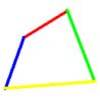#### You may also like### Real(ly) Numbers

If x, y and z are real numbers such that: x + y + z = 5 and xy + yz + zx = 3. What is the largest value that any of the numbers can have?### Biggest Bendy

Four rods are hinged at their ends to form a quadrilateral. How can you maximise its area?### Quartics

Investigate the graphs of y = [1 + (x - t)^2][1 + (x + t^)2] as the parameter t varies.

# Quick Route

##### Age 16 to 18 Challenge Level:

You want to get across a square ploughed field from one corner to the opposite corner as quickly as possible. There is a path along one edge of the field but the rest of the field is ploughed right up to the other three edges. On the ploughed land you can walk at 6 km per hour. From where you are standing you can walk on the path along one edge of the field at 10 km per hour (the path is red in the interactivity below), but there are no paths along the other edges of the field. What is the best route to take?

Suppose the edge of the field is one kilometre in length. What is the shortest time in which you can cross the field to the opposite corner?